Reach Us+441474556909
Fast Approximation Based Combinatorial Optimization Algorithm | OMICS International
Journal of Electrical & Electronic Systems
All submissions of the EM system will be redirected to Online Manuscript Submission System. Authors are requested to submit articles directly to Online Manuscript Submission System of respective journal.

# Fast Approximation Based Combinatorial Optimization Algorithm

Harsimran Kaur1* and Er. Neetu Gupta2

1M.tech Scholar, ECE Department GIMET, Amritsar, Punjab, India

2Assistant Professor, ECE Department, GIMET, Amritsar, Punjab, India

*Corresponding Author:
Harsimran Kaur
M.tech Scholar, ECE Department GIMET
Amritsar, Punjab, India
E-mail: [email protected]

Received Date: September 02, 2014; Accepted Date: December 02, 2014; Published Date: December 15, 2014

Citation: Kaur H, Gupta N (2014) Fast Approximation Based Combinatorial Optimization Algorithm. J Electr Electron Syst 3:135. doi:10.4172/2332-0796.1000135

Copyright: © 2014 Kaur H, et al. This is an open-access article distributed under the terms of the Creative Commons Attribution License, which permits unrestricted use, distribution, and reproduction in any medium, provided the original author and source are credited.

Visit for more related articles at Journal of Electrical & Electronic Systems

#### Abstract

Label cost optimization proposes a new improvement in label cost function, improving existing moves of α-expansion algorithm and introducing some new moves for this algorithm. In order to study the performance comparison, different metrics of energy minimization has been considered. An appropriate comparison has been drawn among proposed technique i.e. fast approximation algorithm and previous well known techniques. The objective is to effectively optimize energies so that satisfactory image segmentation can be obtained (represented with different labels respective to different objects). New combinatorial optimization algorithm have been proposed which shows promising experimental results with the new moves, which we believe could be used in any context where α -expansions are currently employed.

#### Keywords

Energy minimization; Labels; α-expansion; Segmentation; NP-hard

#### Introduction

Energy minimization is of strong practical and theoretical importance to computer vision. Energy expresses our criteria for a good solution—low energies are good, high energies are bad—independent of any algorithm. Algorithms are however hugely important in practice. Even for low level vision problems we are confronted by energies that are computationally hard (often NP-hard) to minimize. As a consequence, a significant portion of computer vision researchis dedicated to identifying energies that are useful and yet reasonably tractable. The work in this paper is of precisely this nature. Computer vision is full of ‘labeling’ problems cost as energy minimization. For example, the data to be labeled could be pixels, interest points, point correspondences, or mesh datasuch as from a range scanner. Depending on the application, the labels could be either semantic (object classes, types of tissue) or describe geometry/appearance (depth, orientation, shape, texture).

Two objective functions known as entropy generation rate and material cost with five constraints have been taken to measure the performance of the heat sink. Number of fins, height of fins, spacing between two fins and oncoming air velocity are considered as the design variables. The dynamic heat dissipation performance of platefin heat sink is investigated using finite element software ANSYS 12.1. The results show the better or competitive performance of the TLBO algorithm over the other optimization algorithms considered by the previous researchers for the same problem.

Labeling problems

A labeling problem is, roughly speaking, the task of assigning an explanatory ‘label’ to each element in a set of observations. Many classical clustering problems are also labeling problems because each data point is assigned a cluster label. To describe a labeling problem one needs a set of observations (the data) and a set of possible explanations. The labeling problem associates one discrete variable with each datum, and the goal is to find the best overall assignment to these variables (a ‘labeling’) according to some criteria. In computer vision, the observations can be things like pixels in an image, salient points within an image, depth measurements from arrange-scanner, or intensity measurements from CT/MRI. The labels are typically either semantic (car, pedestrian, street) or related to scene geometry (depth, orientation, shape, texture).

α-expansion algorithm

The α-expansion algorithm has a significant impact in computer vision due to its generality, effectiveness, and speed. It is commonly used to minimize energies that involve unary, pair wise, and specialized higher-order terms. Their main algorithmic contribution is an extension of α-expansion that also optimizes “label costs” with well characterized optimality bounds. Label costs penalize a solution based on the set of labels that appear in it, for example by simply penalizing the number of labels in the solution. The α-expansion algorithm performs local search using a powerful class of ‘moves’. Given an initial labeling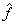and some particular label α ∈ L, an α-expansion move gives each variable the following binary choice: either keep the current label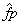, or switch to label α. Let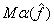denote the set of all moves (labelings) that can be generated this way, in other words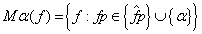All variables are simultaneously allowed to keep their current label or to switch, so there are an exponential number of possible moves. For each choice of α we must efficiently find the best possible move. In practice, this sub-problem is solved by casting it as a graph cut and using combinatorial algorithms to compute the optimal binary configuration. Because a graph cut finds the best move from an exponential number of possibilities, the α-expansion algorithm is a very large-scale neighborhood search (VLSN) technique and is very competitive in practice.

With respect to some current labellingthe full set of possible expansion moves is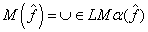.The α-expansion algorithm simply performs local search over the full search neighborhood M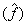.with a labelingthat is within a constant factor from the globally optimal labelling f*.

In HOA, wind parcels move in a spiral course outward from a lowpressure zone called the eye emulating hurricanes in the real world. During this process, wind parcels search for a lower pressure zone (new eye), which is considered as the optimal solution. The HOA is tested with several benchmark functions frequently used in the area of optimization. The obtained results exhibit the high performance of the proposed method.

Different energy minimization algorithms

Iterated conditional modes (ICM): Iterated conditional modes  use a deterministic “greedy” strategy to find a local minimum. It starts with an estimate of the labeling, and then for each pixel it chooses the label giving the largest decrease of the energy function . This process is repeated until convergence, which is guaranteed to occur, and in practice is very rapid. Unfortunately, the results are extremely sensitive to the initial estimate, especiallyin high-dimensional spaces with nonconvex energies (such as arise in vision) due to the huge number of local minima. ICM is assign each pixel the label with the lowest data cost. This resulted in significantly better performance.

Graph cuts: The two most popular graph cuts algorithms , called the swap move algorithm and the expansion move algorithm, were introduced . These algorithms rapidly compute a strong local minimum, in the sense that no “permitted move” will produce a labeling with lower energy. For a pair of labels α, β, a swap move takes some subset of the pixels currently given the label α and assigns them the label β, and vice-versa. The swap movealgorithm finds a local minimum such that there is no swap move, for any pair oflabels α, β that will produce a lower energy labeling. Analogously, we define an expansion move for a label α to increase the set of pixels that are given this label. The expansion move algorithm finds a local minimum such that no expansion move, for any label α, yields a labeling with lower energy. The criteria for a local minimum with respect to expansion moves (swap moves) are so strong that there are many fewer minima in high dimensional spaces compared to standard moves. In the original work of  the swap move algorithm was shown to be applicable to any energy where Vpq is a semi-metric and the expansion move algorithm to any energy where Vpq is a metric. The results of  imply that the expansion move algorithm can be used if for all labels α,β,andγ, Vpq(α, α) + Vpq(β, γ) ≤ Vpq(α, γ) + Vpq(β,α). The swap move algorithm can be used if for all labels α,βVpq(α, α) + Vpq(β, β) ≤ Vpq(α, β) + Vpq(β,α). (This constraint comes from the notion of regular, i.e. submodular, binary energy functions, which are closely related to graph cuts.) If the energy does not obey these constraints, graph cut algorithms can still be applied by “truncating” the violating terms . In this case, however, we are no longer guaranteed to find the optimal labeling with respect to swap (or expansion) moves. In practice, the performance of this version seems to work well when only relatively few terms need to be truncated.

Max-product loopy belief propagation (LBP): To evaluate the performance of LBP, we implemented the max-product LBP version, which is designed to find the lowest energy solution. The other main variant of LBP, the sum-product algorithm, does not directly search for a minimum energy solution, but instead computes the marginal probability distribution of each node in the graph. The belief propagation algorithm was originally designed for graphs without cycles, in which case it produces the exact result for our energy. However, there is nothing in the formulation of BP that prevents it from being tried on graphs with loops. In general, LPB is not guaranteed to converge, and may go into an infinite loop switching between two labeling. Felzenszwalb and Huttenlocher  present a number of ways to speed up the basic algorithm. In particular, LBP implementation uses the distance transform method described in , which significantly reduces the running time of the algorithm.

Tree-reweighted message passing (TRW): Tree-reweighted message passing is a message-passing algorithm similar, on the surface, to LBP. An interesting feature of the TRW algorithm is that it computes a lower bound on the energy. The original TRW algorithm does not necessarily converge, and does not, in fact, guarantee that the lower bound always increases with time. In a research paper an improved version of TRW was used, which is called sequential TRW, or TRW-S. In this version, the lower bound estimate is guaranteed not to decrease, which results in certain convergence properties. In TRW-S we first select an arbitrary pixel ordering function S (p). The messages are updated in order of increasing S (p) and at the next iteration in the reverse order. Trees are constrained to be chains that are monotonic with respect to S (p).

This Introduction covers the terminology and techniques used for the cost labeling approach. Thesis work will be focused around improvement in label cost function, improving existing moves of α-expansion algorithm and introducing some new moves for this algorithm. Some, new technique will be used in α-expansion algorithm to optimize label cost function and utilize it for better results.

#### Related Work

Anton Osokin  in his paper Author describe the α-expansion algorithm has had a significant impact in computer vision due to its generality, effectiveness, and speed. It is commonly used to minimize energies that involve unary, pair wise, and specialized higherorder terms. Their main algorithmic contribution is an extension of α-expansion that also optimizes “label costs” with well characterized optimality bounds. Label costs penalize a solution based on the set of labels that appear in it, for example by simply penalizing the number of labels in the solution. As energy has a natural interpretation as minimizing description length (MDL) and sheds light on classical algorithms like K-means and expectation-maximization (EM). Label costs are useful for multi-model fitting and he demonstrate several such applications: homography detection, motion segmentation, image segmentation and compression.

Lena Gorelick et al.  in this paper author describes computers vision is full of problems elegantly expressed in terms of energy minimization. They characterize a class of energies with hierarchical costs and propose a novel hierarchical fusion algorithm. Hierarchical costs are natural for modeling an array of difficult problems. They explain in example, that in semantic segmentation one could rule out unlikely object combinations using hierarchical context. In geometric model estimation, one could penalize the number of unique model families in a solution, not just the number of models—a kind of hierarchical MDL criterion. Hierarchical fusionuses the well-known α-expansion algorithm as a subroutine, and offers a much better approximation bound in important cases.

Yuri Boykov et al.  in this paper author address the problem of minimizing a large class of energy functions that occur in early vision. The major restriction is that the energy function’s smoothness term must only involve pairs of pixels. He proposes two algorithms that use graph cuts to compute a local minimum even when very large moves are allowed. Thefirst move he consider is an α-β swap: for a pairoflabels α β; this move exchanges the labels between an arbitrary set of pixels labeled and another arbitrary set labeled β. The first algorithm generates a labeling such that there is no swapmove that decreases the energy. The second move he consideredis a α-expansion: for a label α, this move assigns an arbitrary set of pixels the label α. The second algorithm, which requires the smoothness term to be a metric, generates a labeling such that there is no expansion move that decreases the energy. Moreover, this solution is within a known factor of the global minimum. He experimentally demonstrates the effectiveness of his approach on image restoration, stereo and motion.

#### Problem Definition

Image can be segmented by assigning different labels (represented by different colors) to different objects. Label costs penalize a solution based on the set of labels that appear in it, for example by simply penalizing the number of labels in the solution. There should be sufficient number of labels; too many labels do not represent good segmentation as multiple labels may represent subpart of single object. On the other hand, in case of too less number of labels, a single label may represent multiple objects. Label cost can be associated with energy terms (combination of various energies associated with images e.g. Smoothing Energy, Bending Energy, Elastic energy etc.). Most labeling problems in computer vision and machine learning are illposed and in need of regularization, but the most useful regularization algorithms often make the problem NP-hard. The objective is to effectively optimize energies so that satisfactory image segmentation can be obtained (represented with different labels respective to different objects). In order to meet the objective, first task will be to define some label cost function in terms of energies. Unsupervised segmentation will be performed to assign labels by clustering simultaneously over pixels and color space using Gaussian Mixtures (for color images) and nonparametric histograms (for gray-scale images).

Then based upon fast approximation based combinatorial optimization algorithm is implemented to minimize label cost function and redefine labels. α-expansion algorithm is already available for this purpose. This work focused around improvement in label cost function, and incorporating elastic energy for this algorithm.

#### Methodology

Fast approximation based combinatorial optimization algorithm

Label costs: Start by considering a basic (unregularized) energy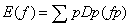, where optimal fp can be determined trivially by minimizing over independent ‘data costs’. We can introduce label costs into E(f) to penalize each unique label that appears in f: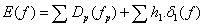Where p∈P l∈L

Minimum graph cut algorithm is performing a graph cut based upon following objective functionf i.e. label, smooth, data and elastic cost.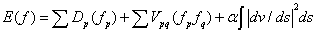p∈P l∈L p,q∈ N 0

It defines how different steps discussed above will be used here to achieve the objectives.

Step 1: Define some label cost function in terms of energies.

Step 2: Unsupervised segmentation will be performed to assign labels by clustering simultaneously over pixels and color space using Gaussian Mixtures (for Color images) and nonparametric histograms (for gray-scale images).

Step 3: Minimum graph cut algorithm is applied to separate two separate layers of the image. Separated layers are added into queue.

Step 4: Repeat until queue is empty.

Step 4a: pop an element from queue.

Step 4b: perform minimum graph cut algorithm.

Step 4c: If selected layer is successfully further separated into sub layer by a minimum graph cut algorithm then add sub-layer to queue elseadd selected layer to the solution list.

Step 5: Assign different Labels/colors to objects present in every single element of solution list [layers].

#### Experimental Results

The experimental setup is essentially the same for each application: generate proposals via random sampling, compute initial data costs Dp, and run the iterative algorithm from the Tables 1 and 2 below compare running times (in seconds, 1.4 GHz Pentium IV) of the selected algorithms for a number of segmentation examples. Note that these times include min-cut/max-flow computation and Fast approximation based combinatorial optimization algorithm (Figures 1-3). In each column we show running times of Fast approximation based combinatorial optimization algorithm and max-flow/min-cut algorithms corresponding to exactly the same set of seeds. The running times were obtained for the “5” and “25” neighborhood systems (N5 and N25). Switching from N5 to N25 increases complexity of graphs but does not affect the quality of segmentation results much.

Methods 2D examples
Bell photo(255x313 Lung CT (409x314) Liver MR(511x511
N4 N8 N4 N8 N4 N8
DINIC 2.73 3.99 2.91 3.45 6.33     22.86
H_PRF 1.27 1.86 1.00 1.22 1.94 2.59
Q_PRF 1.34 0.83 1.17 0.77 1.72 3.45
Combitorial Approach 0.11 0.19 0.32 0.38 0.30 0.55

Table 1: Comparison between running time.

Approximate Methods
Energy minimization case Algorithm Applications
V metric α-expansion  and extensions, LP rounding,r-HST metrics Approximation bounds, segmentation, model fitting
V semi-metric αβ-swap,r-HST metrics Approximation bound
V truncated convex Range moves Approximation bound
¦L¦=2 QPBO ,QPBO bipartite multi-cut Approximation bound α log(#non-submodular terms);QPBO gives partial labelings
Arbitrary energy Mess, passing, decomposition, local search NP-hard to approximate by constant factor

Table 2: Comparisons of Various approximation algorithms results.

#### Conclusion

Different metrics of energy minimization are considered for performance comparison. An appropriate comparison has been drawn among proposed technique and previous well known techniques. The objective is to effectively optimize energies so that satisfactory image segmentation can be obtained (represented with different labels respective to different objects). New combinatorial optimization algorithm have been proposed which shows promising experimental results with the new moves, which we believe could be used in any context where α-expansions are currently employed.

#### References

Select your language of interest to view the total content in your interested language

### Article Usage

• Total views: 12118
• [From(publication date):
November-2014 - Aug 24, 2019]
• Breakdown by view type
• HTML page views : 8324
• PDF downloads : 3794

## Post your commentCan't read the image? click here to refresh
###### Peer Reviewed Journals

Make the best use of Scientific Research and information from our 700 + peer reviewed, Open Access Journals

International Conferences 2019-20

Meet Inspiring Speakers and Experts at our 3000+ Global Annual Meetings

Top